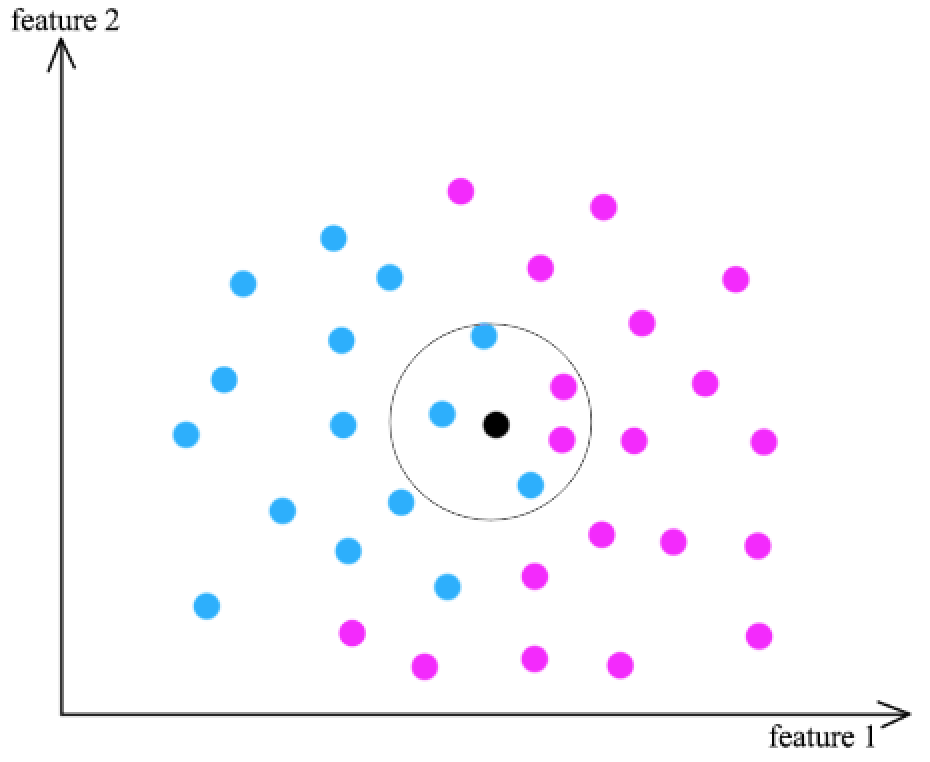# Non-Parametric Model

## What are Non-parametric Models?

Non-parametric Models are statistical models that do not often conform to a normal distribution, as they rely upon continuous data, rather than discrete values. Non-parametric statistics often deal with ordinal numbers, or data that does not have a value as fixed as a discrete number. The term non-parametric does not mean that the value lack inherent parameters, but rather that the parameters are flexible and can vary. One may turn to non-parametric statistics when dealing with ranked data, in which some of the value of the variables is the order in which they are organized.

### Parametric vs. Non-parametric

Parametric statistics are able to infer the traditional measurements associated with normal distributions including mean, median, and mode. While some non-parametric distributions are normally oriented, often one cannot assume the data comes from a normal distribution. For example, a study comparing ranked personal preferences will want to use non-parametric statistics to represent the data as the values aren't going to be normally distributed.

While parametric statistics have a greater degree of certainty about assumptions drawn from the dataset, non-parametric statistics don't need to rely on a mean, median, or mode. Non-parametric statistics make fewer assumptions about the data in a trade-off for decreased accuracy and increased ease of use.

### Non-parametric Models and Machine LearningSource

Non-parametric machine learning algorithms try to make assumptions about the data given the patterns observed from similar instances. For example, a popular non-parametric machine learning algorithm is the K-Nearest Neighbor algorithm that looks at similar training patterns for new instances. The only assumption it makes about the data set is that the training patterns that are the most similar are most likely to have a similar result. While non-parametric machine learning algorithms are often slower and require large amounts of data, they are rather flexible as they minimize the assumptions they make about the data.